# Problems of the area of an annulus

#### Number of problems found: 20

• The collar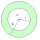The collar on the dress has the shape of an annulus 6 cm wide. The circumference of the inner circle is 31.4 cm. How much cm2 of fabric is needed to make one collar?
• Round skirtThe cut on the round skirt has the shape of an annulus. Determine how much m² of fabric will be consumed on an 80 cm long skirt. The circumference of the waist is a circle with a smaller radius and is 69 cm.
• The hollow cylinderThe hollow cylinder has a height of 70 cm, an outer diameter of 180 cm, and an inner diameter of 120 cm. What is the surface of the body, including the area inside the cavity?
• Annulus from triangleCalculate the content of the area bounded by a circle circumscribed and a circle inscribed by a triangle with sides a = 25mm, b = 29mm, c = 36mm
• Round flowerbed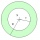Around a round flowerbed with a diameter of 6 meters and I will make a sidewalk up to 0.5 meters wide. How much gravel is needed if the layer is to be 5 cm high?
• Annular areaThe square with side a = 1 is inscribed and circumscribed by circles. Find the annular area.
• Mice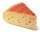Mice consumed a circular hole in a slice of cheese. The cheese has the shape of a circular cut with a radius of 20 cm and an angle of 90 degrees. What percentage of the cheese ate mice if they made 20 holes with a diameter of 2 cm?
• Concentric circlesThere is given a Circle K with a radius r = 8 cm. How large must a radius have a smaller concentric circle that divides the circle K into two parts with the same area?Marie wants to make a cone-shaped witch's hat for a masquerade ball. How much material will it need if it counts on an annular rim with diameters of 28cm and 44cm? Hat side length is 30cm. Add 5% of the material to the bust. Round to cm2.
• Around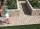Around the circular flowerbed with a radius of 2 m is a sidewalk 80 cm wide. How many square meters does the sidewalk have?
• The coilHow many ropes (the diameter 8 mm) fit on the coil (threads are wrapped close together) The coil has dimension: the inner diameter 400mm, the outside diameter 800mm and the length of the coil is 470mm
• Two annulusesThe area of the annular circle formed by two circles with a common center is 100 cm2. The radius of the outer circle is equal to twice the radius of the inner circle. Determine the outside circle radius in centimeters.
• Pipe cross sectionThe pipe has an outside diameter 1100 mm and the pipe wall is 100 mm thick. Calculate the cross section of this pipe.
• AnnulusThe radius of the larger circle is 8cm, the radius of smaller is 5cm. Calculate the contents of the annulus.
• Circular ringSquare with area 16 centimeters square are inscribed circle k1 and described circle k2. Calculate the area of circular ring, which circles k1, k2 form.
• Annulus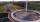Calculate the area of two circles annulus k1 (S, 3 cm) and k2 (S, 5 cm).
• SidewalkThe city park is a circular bed of flowers with a diameter of 8 meters. Around it, the whole length is 1-meter wide sidewalk. What is the sidewalk area?
• Annulus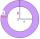Two concentric circles form an annulus of width 10 cm. The radius of the smaller circle is 20 cm. Calculate the content area of the annulus.
• Circular lawnAround a circular lawn area is 2 m wide sidewalk. The outer edge of the sidewalk is curb whose width is 2 m. Curbstone and the inner side of the sidewalk together form a concentric circles. Calculate the area of the circular lawn and the result round to 1
• Circular flowerbed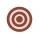Circular flowerbed with diameter 8 m we split by concentric circle to circle and annulus with the same area. Find the radius of the circle.

Do you have an exciting math question or word problem that you can't solve? Ask a question or post a math problem, and we can try to solve it.

We will send a solution to your e-mail address. Solved examples are also published here. Please enter the e-mail correctly and check whether you don't have a full mailbox.

Annulus Problems. Area - math problems.